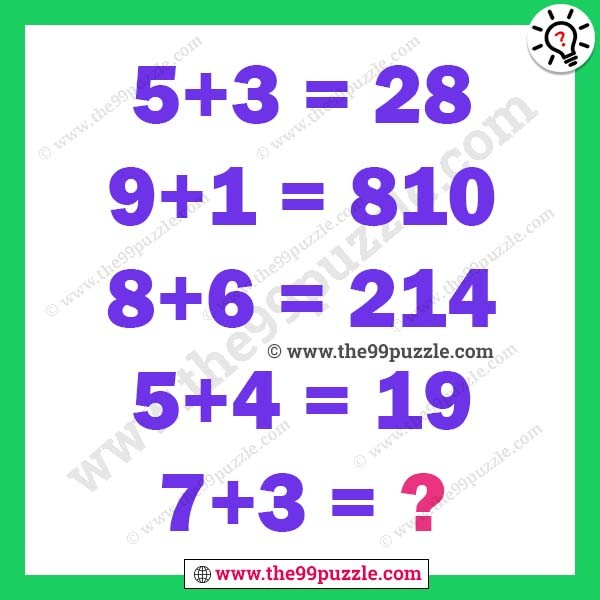# Mathematical logic question for adults – Puzz215

This mathematical logic question is for adults and teens. Can you solve this math reasoning puzzle? Always trying to solve this type of brain teaser math puzzle. You learn maths shortcut tricks and math logic from it. Sometimes this math puzzle gets very hard but when you crack the math logic this is very easy to solve. In this math equation puzzle, some equations give here. All equations are in the same math logic. You have to crack the logic and solve the last equation.

5+3=28

9+1=810

8+6=214

5+4=19

7+3=?###### Explanation:

5+3 = (5-3)  (5+3) = 28

9+1 = (9-1) (9+1) = 810

8+6 = (8-6) (8+6) = 214

5+4= (5-4) (5+4) = 19

7+3= (7-3) (7+3) = 410